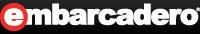Articles Members Online: 3
 -Article/Tip Search -News Group-Delphi/Pascal -CBuilder/C++ -C#Builder/C# -JBuilder/Java -Kylix Member Area -Home -Account Center -Top 10 NEW!! -Submit Article/Tip -Forums Upgraded!! -My Articles -Edit Information -Login/Logout -Become a Member -Why sign up! -Newsletter -Chat Online! -Indexes NEW!! Employment -Build your resume -Find a job -Post a job -Resume Search Contacts -Contacts -Feedbacks -Link to us -Privacy/Disclaimer
 EmbarcaderoEmbarcadero Community JEDI Links
 27-May-03
 Category ActiveX Language Delphi 3.x Views 124 User Rating No Votes # Votes 0 Replies 0
 Publisher: DSP, Administrator Reference URL: DKB
 ``` Author: Ernesto De Spirito How can I round a number "normally"? Can I round the thousands? Can I round the third digit after the decimal point? Answer: Integer rounding The Round function that comes with Delphi performs what is called "banker's rounding", meaning that a number with a fractional part of 0.5 is rounded sometimes up and sometimes down, always towards the nearest even number. This means that for example Round(3.5) gives 4 while Round(2.5) gives 2. Here goes a set of functions for rounding numbers, including RoundN which rounds a number "normally" (i.e. RoundN(3.5) is 4 and RoundN(2.5) is 3). 1 function Sgn(X: Extended): Integer; 2 // Returns -1, 0 or 1 according to the 3 // sign of the argument 4 begin 5 if X < 0 then 6 Result := -1 7 else if X = 0 then 8 Result := 0 9 else 10 Result := 1; 11 end; 12 13 function RoundUp(X: Extended): Extended; 14 // Returns the first integer greater than or 15 // equal to a given number in absolute value 16 // (sign is preserved). 17 // RoundUp(3.3) = 4 RoundUp(-3.3) = -4 18 begin 19 Result := Int(X) + Sgn(Frac(X)); 20 end; 21 22 function RoundDn(X: Extended): Extended; 23 // Returns the first integer less than or 24 // equal to a given number in absolute 25 // value (sign is preserved). 26 // RoundDn(3.7) = 3 RoundDn(-3.7) = -3 27 begin 28 Result := Int(X); 29 end; 30 31 function RoundN(X: Extended): Extended; 32 // Rounds a number "normally": if the fractional 33 // part is >= 0.5 the number is rounded up (see RoundUp) 34 // Otherwise, if the fractional part is < 0.5, the 35 // number is rounded down (see RoundDn). 36 // RoundN(3.5) = 4 RoundN(-3.5) = -4 37 // RoundN(3.1) = 3 RoundN(-3.1) = -3 38 begin 39 (* 40 if Abs(Frac(X)) >= 0.5 then 41 Result := RoundUp(X) 42 else 43 Result := RoundDn(X); 44 *) 45 Result := Int(X) + Int(Frac(X) * 2); 46 end; 47 48 function Fix(X: Extended): Extended; 49 // Returns the first integer less than or 50 // equal to a given number. 51 // Int(3.7) = 3 Int(-3.7) = -3 52 // Fix(3.7) = 3 Fix(-3.1) = -4 53 begin 54 if (X >= 0) or (Frac(X) = 0) then 55 Result := Int(X) 56 else 57 Result := Int(X) - 1; 58 end; 59 60 function RoundDnX(X: Extended): Extended; 61 // Returns the first integer less than or 62 // equal to a given number. 63 // RoundDnX(3.7) = 3 RoundDnX(-3.7) = -3 64 // RoundDnX(3.7) = 3 RoundDnX(-3.1) = -4 65 begin 66 Result := Fix(X); 67 end; 68 69 function RoundUpX(X: Extended): Extended; 70 // Returns the first integer greater than or 71 // equal to a given number. 72 // RoundUpX(3.1) = 4 RoundUpX(-3.7) = -3 73 begin 74 Result := Fix(X) + Abs(Sgn(Frac(X))) 75 end; 76 77 function RoundX(X: Extended): Extended; 78 // Rounds a number "normally", but taking the sign into 79 // account: if the fractional part is >= 0.5 the number 80 // is rounded up (see RoundUpX) 81 // Otherwise, if the fractional part is < 0.5, the 82 // number is rounded down (see RoundDnX). 83 // RoundX(3.5) = 4 RoundX(-3.5) = -3 84 begin 85 (* 86 if Abs(Frac(X)) >= 0.5 then 87 Result := RoundUpX(X) 88 else 89 Result := RoundDnX(X); 90 *) 91 Result := Fix(X + 0.5); 92 end; Rounding to a decimal digit The functions we've just presented above always round to the last integer digit, but sometimes we need to round for example to the second decimal or to the thousands, millions or billions. You can overload the RoundN function with this version that takes an extra parameter to indicate the digit to be round: 93 function RoundN(x: Extended; d: Integer): Extended; 94 // RoundN(123.456, 0) = 123.00 95 // RoundN(123.456, 2) = 123.46 96 // RoundN(123456, -3) = 123000 97 const 98 t: array[0..12] of int64 = (1, 10, 100, 1000, 10000, 100000, 99 1000000, 10000000, 100000000, 1000000000, 10000000000, 100 100000000000, 1000000000000); 101 begin 102 if Abs(d) > 12 then 103 raise ERangeError.Create('RoundN: Value must be in -12..12'); 104 if d = 0 then 105 Result := Int(x) + Int(Frac(x) * 2) 106 else if d > 0 then 107 begin 108 x := x * t[d]; 109 Result := (Int(x) + Int(Frac(x) * 2)) / t[d]; 110 end 111 else 112 begin // d < 0 113 x := x / t[-d]; 114 Result := (Int(x) + Int(Frac(x) * 2)) * t[-d]; 115 end; 116 end; Copyright (c) 2001 Ernesto De Spiritomailto:edspirito@latiumsoftware.com Visit: http://www.latiumsoftware.com/delphi-newsletter.php ```